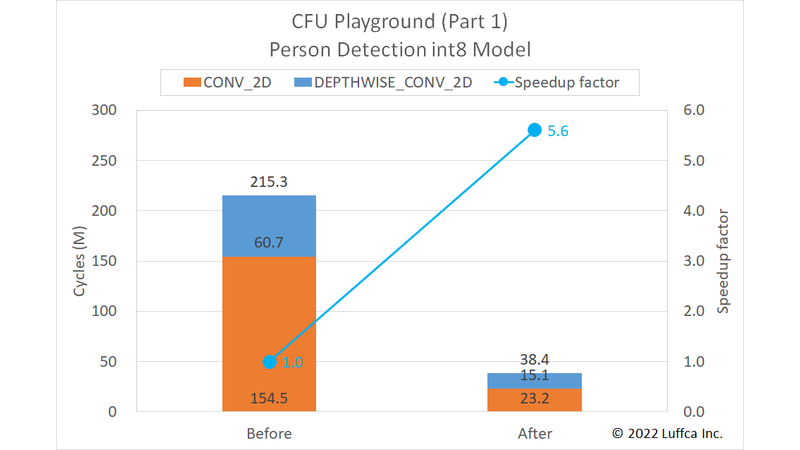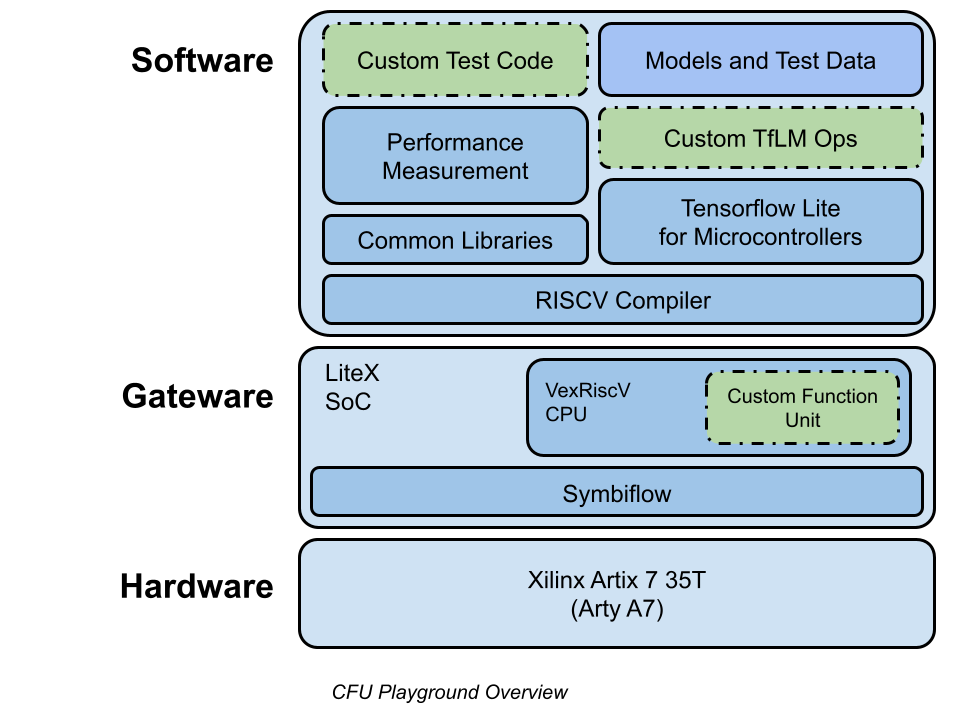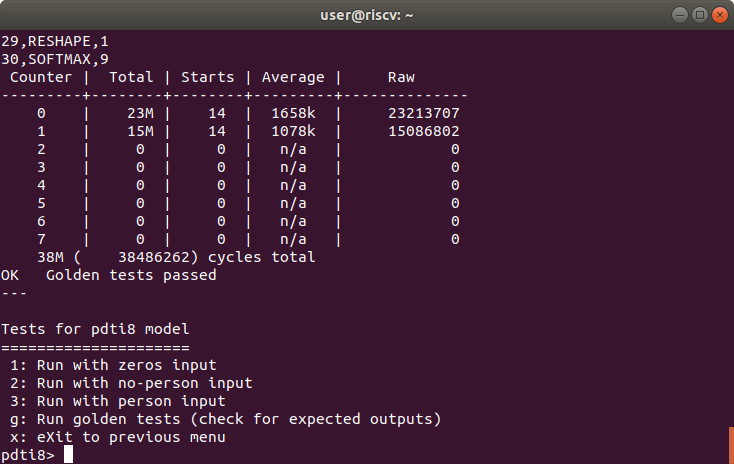# Building an ML Processor using CFU Playground (Part 1)We have built a machine learning (ML) processor on an Arty A7-35T using CFU Playground. In Part 1, we have accelerated the inference of the Person Detection int8 model by 5.6 times.

Note: Part 1 was updated on 8/21/2022 to reflect the results of Part 3.

## CFU Playground

CFU Playground is a framework for tuning hardware (actually FPGA gateware) and software to accelerate the ML model of Google’s TensorFlow Lite for Microcontrollers (TFLM).
The figure below, quoting cfu-playground.readthedocs.io, gives an overview of the CFU Playground.CFU Playground utilizes RISC-V custom instructions to accelerate the inference of the ML model.
To add hardware for custom instructions, it uses a feature called Custom Function Unit (CFU) provided by the combination of RISC-V 32-bit soft CPU VexRiscv and SoC Builder LiteX.
For detail, it uses Amaranth HDL (previously nMigen) to generate `cfu.v` from Python code and combine it with VexRiscv and LiteX Verilog files to create gateware. You can also create gateware by creating `cfu.v` directly.
On the software side, `#include "cfu.h"` allows the custom instruction `cfu_op(funct3, funct7, rs1, rs2)` to be used within the TFLM.
In addition, there is a mechanism to measure the number of cycles of a specific process by using a performance counter. However, it seems that it may not be available on FPGA boards with limited resources.

## Person Detection int8 Model

The Person Detection int8 model, called `pdti8` in CFU Playground, is also featured in The Step-by-Step Guide to Building an ML Accelerator (hereafter Guide).
This model is a total of 31 layers of `CONV_2D` and `DEPTHWISE_CONV_2D` (hereinafter `dw_conv`) with 14 layers each and `AVERAGE_POOL_2D`, `RESHAPE`, and `SOFTMAX` with 1 layer each.
The `CONV_2D` in this model actually works as a 1×1 convolution (hereafter `1x1_conv`).

## Building an ML ProcessorResult of golden tests for pdti8 model

In the Guide, the target of acceleration is only `1x1_conv`, but this time we also targeted `dw_conv`.
Introducing the results first, we were able to speed up the total number of cycles of the `pdti8` model from 215.3M to 38.4M, as shown in the featured image and the table below.

Person Detection int8 Model Cycles Speedup
factor
Before After
`1x1_conv` 154.5M 23.2M 6.7
`dw_conv` 60.7M 15.1M 4.0
Total 215.3M 38.4M 5.6

In the Guide, the total number of cycles after acceleration is 86M, so it was possible to accelerate compared to this.
Also, when we previously benchmarked the GitHub `proj/avg_pdti8` that is accelerating the `pdti8` model, the total number of cycles was 67M, so the acceleration of `dw_conv` contributed to the result.

### Software Specialization

As described in the Guide, software specializations are effective in accelerating the ML model.

The `ConvPerChannel` function of `CONV_2D` looks like this:

```for (int batch = 0; batch < batches; ++batch) {
for (int out_y = 0; out_y < output_height; ++out_y) {
const int in_y_origin = (out_y * stride_height) - pad_height;
for (int out_x = 0; out_x < output_width; ++out_x) {
const int in_x_origin = (out_x * stride_width) - pad_width;
for (int out_channel = 0; out_channel < output_depth; ++out_channel) {
int32_t acc = 0;
for (int filter_y = 0; filter_y < filter_height; ++filter_y) {
const int in_y = in_y_origin + dilation_height_factor * filter_y;
for (int filter_x = 0; filter_x < filter_width; ++filter_x) {
const int in_x = in_x_origin + dilation_width_factor * filter_x;

// Zero padding by omitting the areas outside the image.
const bool is_point_inside_image =
(in_x >= 0) && (in_x < input_width) && (in_y >= 0) &&
(in_y < input_height);

if (!is_point_inside_image) {
continue;
}

for (int in_channel = 0; in_channel < input_depth; ++in_channel) {
...
```

In the `ConvPerChannel1x1` function specialized for `1x1_conv`, the filter size is 1×1, so loops and variables can be omitted, and the calculation of `is_point_inside_image` is not required, so the code is as follows.

```for (int batch = 0; batch < batches; ++batch) {
for (int y = 0; y < output_height; ++y) {
for (int x = 0; x < output_width; ++x) {
for (int out_channel = 0; out_channel < output_depth; ++out_channel) {
int32_t acc = 0;
for (int in_channel = 0; in_channel < input_depth; ++in_channel) {
...
```

### CFU Optimization

As an example of CFU optimization, a `SIMD multiply-accumulate (MAC)` custom instruction introduced in the Guide has the following input/output.

```              int8_t           int8_t           int8_t           int8_t
+----------------+----------------+----------------+----------------+
in0 = | input_data  | input_data  | input_data  | input_data  |
+----------------+----------------+----------------+----------------+

int8_t           int8_t           int8_t           int8_t
+----------------+----------------+----------------+----------------+
in1 = | filter_data | filter_data | filter_data | filter_data |
+----------------+----------------+----------------+----------------+

int32_t
+-------------------------------------------------------------------+
output = | output + (input_data[0, 1, 2, 3] + 128) * filter_data[0, 1, 2, 3] |
+-------------------------------------------------------------------+
```

In addition, `funct3` and `funct7` are assigned to 2 and 1, respectively.

The inner loop of the original `ConvPerChannel` function looks like this:

```for (int in_channel = 0; in_channel < input_depth; ++in_channel) {
int32_t input_val = input_data[Offset(
input_shape, batch, in_y, in_x, in_channel)];
int32_t filter_val = filter_data[Offset(
filter_shape, out_channel, filter_y, filter_x, in_channel)];
acc += filter_val * (input_val + input_offset);
}
```

The inner loop of the `ConvPerChannel1x1` function specialized for `1x1_conv` corresponding to the above is as follows.

```for (int in_channel = 0; in_channel < input_depth; in_channel += 4) {
uint32_t input_val_4 = *((uint32_t*)(input_data + Offset(
input_shape, batch, y, x, in_channel)));
uint32_t filter_val_4 = *((uint32_t*)(filter_data + Offset(
filter_shape, out_channel, 0, 0, in_channel)));
acc = cfu_op2(1, input_val_4, filter_val_4);
}
```

In the case of `1x1_conv`, since TFLM is the channel last, `input_data` and `filter_data` of `int8_t` can be accessed consecutively.

## Summary

We have built an ML processor on an Arty A7-35T using CFU Playground. The ML processor can perform inference of the Person Detection int8 model in 38.4M cycles.

Previous article

Next article